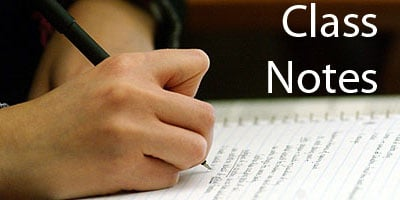## Maths Class 10 Notes for Surface Areas and Volumes

(A) RIGHT CIRCULAR CYLINDER:

A right circular cylinder is solid generated by the revolution of a rectangle about of its sides.

NOTE : If a paper, cylinder open at both the ends is cut along a vertical line on the curved surface and stretched on a plane surface, we obtain a rectangle of length i.e., 27πr and breadth= Height of cylinder h.
So, curved surface area (C.S.A) or lateral surface area = 2πr * height

Important Formula For Cylinder

1. C. S. A of cylinder = ( Perimeter of base) * Height = 2πrh2. Area of each end of cylinder = 2πr2

3. Total surface area (including both circular ends) = 2πrh + 2πr2 = 27πr(h + r)

4. Volume of cylinder — πr2h = [(Area of base) * height]

Hollow Cylinder’s formulae e.g., (Rubber tubes pipes, etc.)

1. Volume of material = Exterior volume — Interior
volume = πR2h — πr2h = πh(R2 – r2)

2. C. S. A or L. S. A = external surface area + internal surface area= 2πRh + 2πrh

3. T. S . A. of hollow cylinder = C. S. A+ 2 ( area of base ring )

= (2πRh + 2πrh) + 2(πR2 – πr2)

NOTE:

1. Two end faces of right circular cylinder are circles having each area = πr2

2. Mass of cylinder = Volume * density

3. When rectangular sheet of paper is rolled along its length , we get a cylinder whose base circumference is length of sheet and height is same as breadth of sheet.

(B) CONE

From figure, AO = height of cone and is denoted by ‘h’OB = radius of the base of cone, AB = slant height of a cone (l)

Important Formula Of rt. Circular Cone :

1. Volume of cone = 1 / 3 πr2h

2 C. S. A or L. S. A=πrl where slant height

= l =√ r2 + hr2

3. T. S. A of cone = πrl + πr2

(C) FRUSTUM OF A CONE

FRUSTUM : A cone is cut by a plane parallel to the base of the cone,then the portion between the plane and base is called frustum of the cone

Important Formulae for Frustum :

1. Volume of frustum of cone
= πh / 3[R2 + r2 + Rr] cubic unit

2. L. S. A or C. S. A = πl(R + r) Sq units where l2 = h2 + (R – r)2

3. T. S. A = πR2 + πr2 + πl(R + r) Sq. units.
(Area of base + Area of top + Area of lateral )

4. Slant height (l) = √h22 + (R – r)2

(D) IMPORTANT FORMULA FOR SPHERE AND HEW-SPHERE

(a) Surface area of sphere = 4πr2(b) Volume of sphere = 4 / 3 πr3

(c) Volume of hemisphere = 2 / 3 πr3

(d) C.S.A. of hemisphere = 2πr2

(e) Total surface area of Hemi-sphere = 2πr2 + πr2 =3πr2

(E) IMPORTANT FORMULA FUR SPHERICAL SHELL/ HEMILSPHERICAL SHELL

(a) Outer surface area of spherical shell =4πR2

(b) Inner S.A. of spherical shell = 4πr2

(c) Total surface area of spherical shell = 4π(R2+ r2)

(d) Volume of spherical shell of external radius R and internal

radius ‘r’ = 4 / 3π(R3 – r3)

(e) Outer curved surface area hemispherical shell = 2πR2(f) Inner curved surface area of hemispherical shell = 2πr2

(g) Thick hemispherical bowl of external and internal radii R and r,

Total S.A. = π(3R2+ r2)

(h) Volume of hemispherical shell of external radius ‘R’ and internal radius ‘r’

= 2 / 3π(R3 — r2).